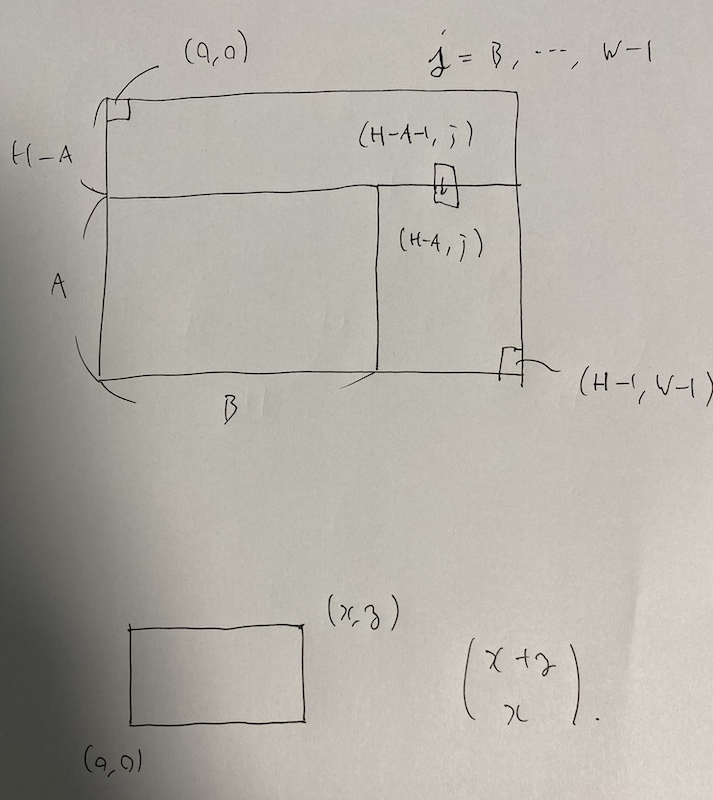# AtCoder Regular Contest 058

Updated:

AtCoder Regular Contest 058

• Review: 2020-07-06

# Solutions

## C - こだわり者いろはちゃん / Iroha’s Obsession

Just try by $n, n + 1, \dots$. Since $n \leq 10 ^ 4$, we can stop before $20000$.

## D - いろはちゃんとマス目 / Iroha and a Grid

Every path passes through $(h - a - 1, j) \mapsto (h - a, j)$. This $j \in [b, w)$ is uniquely exists for every path. Thus, the answer is $\sum _ {j = b} ^ {w - 1} \begin{pmatrix} (h - a - 1) + j \\ h - a - 1 \end{pmatrix} \begin{pmatrix} (h - 1 - (h - a)) + (w - 1 - j) \\ (h - 1 - (h - a)) \end{pmatrix}.$## E - 和風いろはちゃん / Iroha and Haiku

Assume $0$-indexed.

### How to preserve the last terms

We count the sequence which does not contain any XYZ.

We use bitwise memory. We describe that the previous term is $k$ by 1 << k. For example, we mean that the last $3$ terms are $2, 3, 1$ by $101001 _ {(2)}$. We convert $\{ a _ i \}$ into $bit$ in this way. Then, we see the following properties.

•  Judge of XYZ: We define $haiku = (1 \mathbin{«} (Z - 1)) (1 \mathbin{«} (Z + Y - 1) (1 \mathbin{«} (Z + Y + X - 1)))$, and we determine $bit$ has XYZ if $bit \& haiku = haiku$.
• The way of preserving the last terms: We possess $bit \& mask$, where $mask = ((1 \mathbin{«} X + Y + Z - 1) - 1)$. In other words, we save last $X + Y + Z - 1$ bits.

### DP part

#### Definition

vector<vector<mint>> $dp[i][j] =$ the number of the sequences which does not contain any XYZ, where the last terms are described by $j \subset B$.

#### Initial state

$dp = 1$; otherwise $0$.

#### Answer

$10 ^ n - \sum _ {j \subset mask} dp[n][j].$

#### Transition

For $i = 0, \dots, n - 0$, for $j \subset mask$, we execute as follows. If $dp[i][j] = 0$, continue;. Otherwise, for $k = 1, \dots, 10$, let $nj = (j \mathbin{«} k) | (1 \mathbin{«} (k - 1)).$ If $nj$ contains $haiku$, continue;. Otherwise, we execute the following line. $dp[i + 1][nj \& mask] \mathbin{+=} dp[i][j].$

Tags:

Categories: